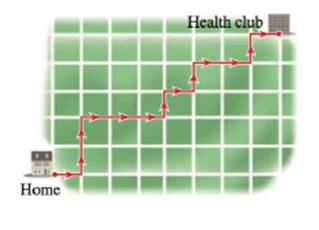Chapter 14.1, Problem 74E### Algebra and Trigonometry (MindTap ...

4th Edition
James Stewart + 2 others
ISBN: 9781305071742

#### Solutions

Chapter
Section### Algebra and Trigonometry (MindTap ...

4th Edition
James Stewart + 2 others
ISBN: 9781305071742
Textbook Problem

# APPLICATIONS61-74 ■ Combinations These exercises involve counting combinations.Jogging Routes A jogger jogs every morning to his health club, which is eight blocks east and five blocks north of his home. He always takes a route that is as short as possible, but he likes to vary it (see figure). How many different routes can he take ? [Hint: The route shown can be thought of as ENNEEENENEENE, where E is East and N is North.]To determine

To find:

The number of different routes the jogger can take to reach the health club.

Explanation

Given:

The jogger has to jogs 13 blocks; 8 East and 5 North, in every possible combination.

Approach:

First choose 8 out of 13 blocks east and then the 5 out of 13 then that would give the desired result.

The formula of the number of combinations as follows.

C(n,r)=n!r!(nr)!(1)

Here, n is the total number of objects and r is the number of objects chosen.

Calculation:

First, substitute 13 for n and 8 for r in equation (1) as.

C(13,8)=13!8!(138)!=13×12×11×10×9×8

### Still sussing out bartleby?

Check out a sample textbook solution.

See a sample solution

#### The Solution to Your Study Problems

Bartleby provides explanations to thousands of textbook problems written by our experts, many with advanced degrees!

Get Started